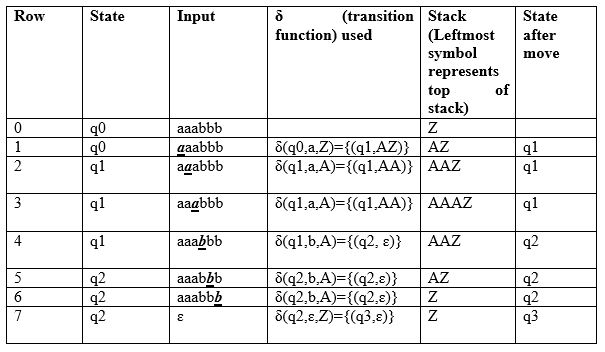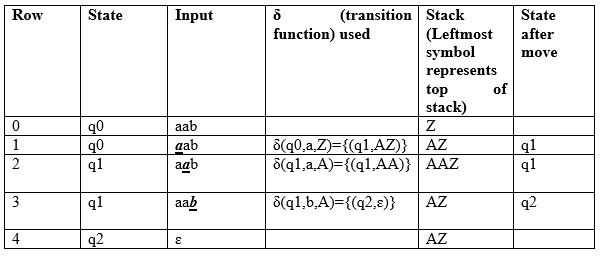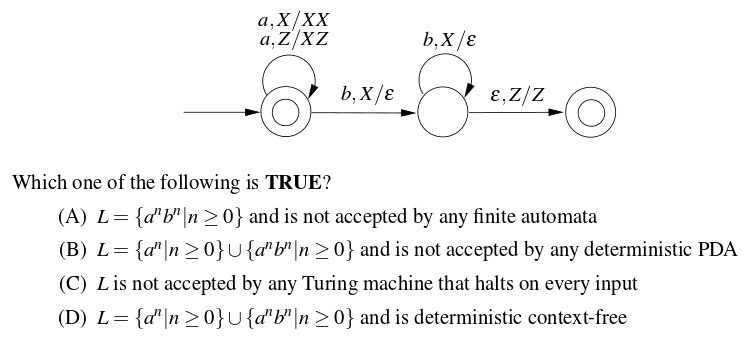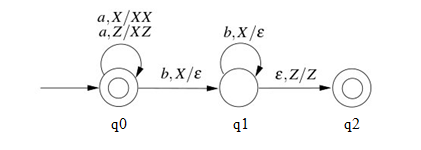Open in App
Not now

# Pushdown Automata Acceptance by Final State

• Difficulty Level : Easy
• Last Updated : 30 May, 2022

We have discussed Pushdown Automata (PDA) and its acceptance by empty stack article. Now, in this article, we will discuss how PDA can accept a CFL based on the final state. Given a PDA P as:

`P = (Q, Σ, Γ, δ, q0, Z, F)`

The language accepted by P is the set of all strings consuming which PDA can move from initial state to final state irrespective of any symbol left on the stack which can be depicted as:

`L(P) = {w |(q0, w, Z) =>(qf, ɛ, s)}`

Here, from start state q0 and stack symbol Z, the final state qf ɛ F is reached when input w is consumed. The stack can contain a string s which is irrelevant as the final state is reached and w will be accepted.

Example: Define the pushdown automata for language {a^nb^n | n > 0} using final state.

Solution: M = where Q = {q0, q1, q2, q3} and ∑ = {a, b} and Γ = { A, Z } and F={q3} and δ is given by:

```δ( q0, a, Z ) = { ( q1, AZ ) }
δ( q1, a, A) = { ( q1, AA ) }
δ( q1, b, A) = { ( q2, ɛ) }
δ( q2, b, A) = { ( q2, ɛ) }
δ( q2, ɛ, Z) = { ( q3, Z) }```

Let us see how this automaton works for aaabbb:Explanation: Initially, the state of automata is q0 and symbol on the stack is Z and the input is aaabbb as shown in row 0. On reading a (shown in bold in row 1), the state will be changed to q1 and it will push symbol A on the stack. On next a (shown in row 2), it will push another symbol A on the stack and remain in state q1. After reading 3 a’s, the stack will be AAAZ with A on the top. After reading b (as shown in row 4), it will pop A and move to state q2 and the stack will be AAZ. When all b’s are read, the state will be q2 and the stack will be Z. In row 7, on input symbol ɛ and Z on the stack, it will move to q3. As the final state q3 has been reached after processing input, the string will be accepted. This type of acceptance is known as acceptance by the final state. Next, we will see how this automata works for aab:As we can see in row 4, the input has been processed and PDA is in state q2 which is a non-final state, the string aab will not be accepted. Let us discuss the question based on this:

Que-1. Consider the transition diagram of a PDA given below with input alphabet ∑ = {a, b}and stack alphabet Γ = {X, Z}. Z is the initial stack symbol. Let L denote the language accepted by the PDA. (GATE-CS-2016)Solution: We first label the state of the given PDA as:Next, the given PDA P can be written as:

``` Q = {q0, q1, q2} and ∑ = {a, b}
And Γ = {X, Z} and F={q0,q2} and δ is given by :
δ( q0, a, Z ) = {( q0, XZ)}
δ( q0, a, X) = {( q0, XX )}
δ( q0, b, X) = {( q1, ɛ)}
δ( q1, b, X) = {( q1, ɛ)}
δ( q1, ɛ, Z) = {( q2, Z)}```

As we can see, q0 is the initial as well as the final state, ɛ will be accepted. For every a, X is pushed onto the stack and PDA remains in the final state. Therefore, any number of a’s can be accepted by PDA. If the input contains b, X is popped from the stack for every b. Then PDA is moved to the final state if the stack becomes empty after processing input (δ( q1, ɛ, Z) = {( q2, Z)}). Therefore, a number of b must be equal to the number of an if they exist. As there is only one move for a given state and input, the PDA is deterministic. So, the correct option is (D).

My Personal Notes arrow_drop_up
Related Articles